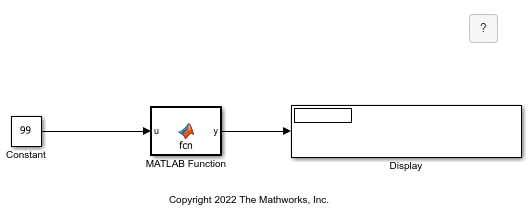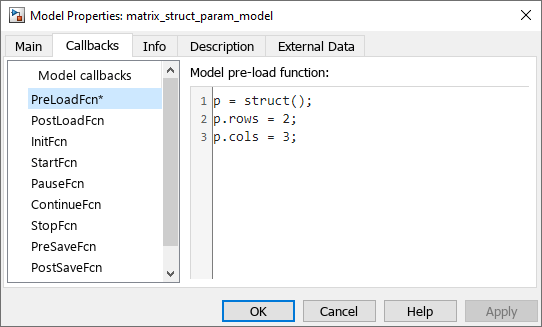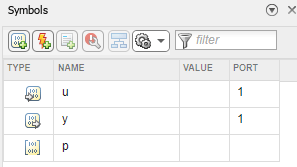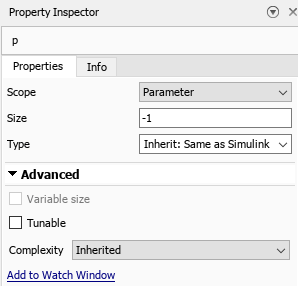# Initialize Matrix Using a Nontunable Structure Parameter

This example shows how to use a nontunable structure parameter input to output a matrix in a MATLAB Function block. Open the model, `matrix_struct_param_model`.In this example, the model uses a pre-load callback that defines a structure, `p`, in the base workspace. `p` has two fields, `rows` and `cols`. To view the callback, in the Modeling tab, click Model Settings > Model Properties. In the Callbacks tab, click PreLoadFcn.### View the MATLAB Function Block and Variable Properties

Open the MATLAB Function block. The function uses the block input `u` and the parameter `p` to define the matrix output `y`. The function adds the value of the input variable `u` to each element of an empty matrix with a size determined by the fields of `p`. The function uses this MATLAB code:

```function y = fcn(u, p) y = zeros(p.rows,p.cols) + u; ```

In the Function tab, click Edit Data to open the Property Inspector and the Symbols pane. `p` is a parameter variable, and therefore does not have a port. See Configure MATLAB Function Block Parameter Variables. Instead, it uses the values from the base workspace variable `p` defined in the callback.Click `p` to view the properties in the Property Inspector. The Scope property of `p` is `Parameter` and the Tunable property is cleared.### Run the Model

Run the model. The MATLAB Function block outputs a 2-by-3 matrix with `99` in each element. You can adjust the size of the matrix by adjusting the fields of `p` in the callback.